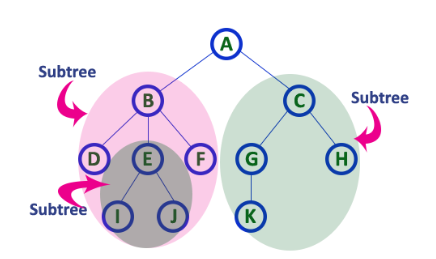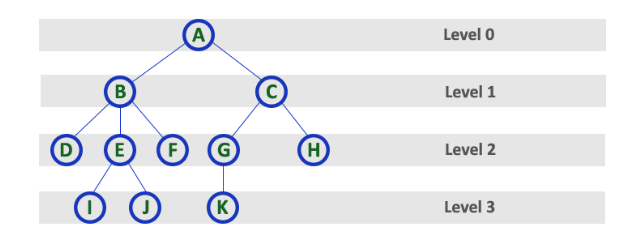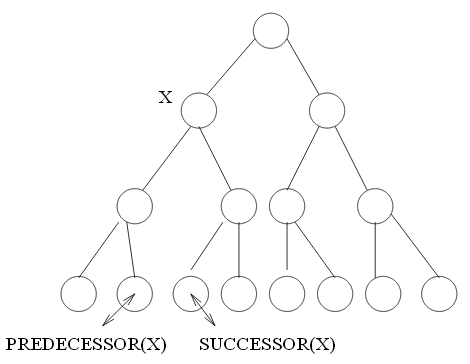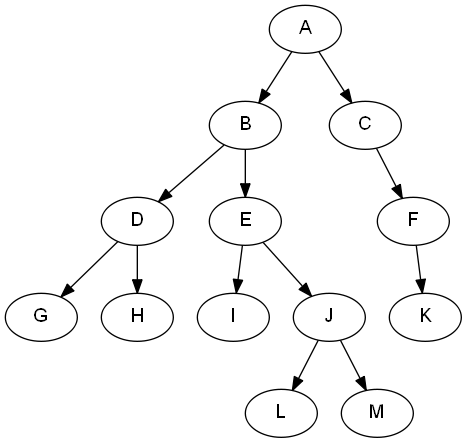# Tree Terminology

## Terminology

Like "list" in Chapter 3, "Trees" is another type of abstraction.

tree, root, edge

We can define a tree recursively. A tree is a collection of nodes. The collection can be empty; otherwise, a tree consists of a distinguish node r, called the root, and zero or more nonempty (sub)tress $$T_1$$, $$T_2$$, ..., $$T_k$$, each of whose roots are connected by a directed edge from r.child, parent

The root of each subtree is said to be a child of r, and r is the parent of each subtree root.

leaves

Nodes with no children are known as leaves.

siblings

Nodes with the same parent are siblings.

path

A path from node $$n_1$$ to $$n_k$$ is defined as a sequence of nodes $$n_1$$, $$n_2$$, ..., $$n_k$$ such that $$n_i$$ is the parent of $$n_{i+1}$$ for $$1<= i < k$$.

length

The length of this path is the number of edges on the path, namely $$k-1$$.

Note

• There is a path of length zero from every node to itself.
• Notice that in a tree there is exactly one path from the root to each node.

depth

For any node $$n_i$$, the depth of $$n_i$$, is the length of the unique path from the root to $$n_i$$.

internal path length

The sum of the depths of all nodes in a tree is known as the internal path length.

height

The height of $$n_i$$ is the length of the longest path from $$n_i$$ to a leaf. (i.e., the height of a node is the number of edges from the node to the deepest leaf)

ancestor, descendant

If there is a path from $$n_1$$ to $$n_2$$, then $$n_1$$ is an ancestor of $$n_2$$ and $$n_2$$ is a descendant of $$n_1$$. If $$n_1 \neq n_2$$, then $$n_1$$ is a proper ancestor of $$n_2$$ and $$n_2$$ is a proper descendant of $$n_1$$.

internal node

An internal node is a node with at least one child. In other words, internal nodes are nodes other than leaves.

degree

The total number of children of a node is called as degree of that node. The highest degree of a node among all the nodes in a tree is called as degree of tree.

level

The root node is said to be at level 0 and the children of root node are at level 1 and the children of the nodes which are at level 1 will be at level 2 and so on ... In other words, in a tree each step from top to bottom is called as a level and the level count starts with '0' and incremented by one at each level (step).predecessor / successor

If $$X$$ has two children, its predecessor is the maximum value in its left subtree and its successor the minimum value in its right subtree. It makes sense if we do in-order traversal of the tree.Inorder traversal

Given a tree shown below, the inorder traversal (left, root, right) gives: 4, 2, 5, 1, 3Preorder traversal

Given the same tree above, the preorder traversal (root, left, right) gives: 1, 2, 4, 5, 3

Postorder traversal

Given the same tree above, the postorder traversal (left, right, root) gives: 4, 5, 2, 3, 1

## Some properties

• A tree is a collection of N nodes, one of which is the root, and N-1 edges. (since each edge connects some node to its parent, and every node except the root has one parent.)
• The root is at depth 0.
• All leaves are at height 0.
• The height of a tree is equal to the height of the root.
• The depth of a tree is equal to the depth of the deepest leaf; this is always equal to the height of the tree.

## Example

Let's work through MAW 4.1, 4.2, and 4.3 to get the tree terminology clear.• "A" is the root
• "G", "H", "I", "L", "M", "K" are the leaves
• "A":
• children: "B", "C"
• depth: 0
• height: 4
• "B":
• parent: "A"
• children: "D", "E"
• siblings: "C"
• depth: 1
• height: 3
• The depth of the tree is 4